Select Page

# 12 Science Maths CBSE Answers for MCQ Vector Algebra in English

12 Science Maths CBSE Answers for MCQ Vector Algebra in English to enable students to get Answers in a narrative video format for the specific question.

Expert Teacher provides 12 Science Maths CBSE Answers for MCQ Vector Algebra through Video Answers in English language. This video solution will be useful for students to understand how to write an answer in exam in order to score more marks. This teacher uses a narrative style for a question from Vector Algebra not only to explain the proper method of answering question, but deriving right answer too.

Please find the question below and view the Answer in a narrative video format.

Question:

## Similar Questions from CBSE, 12th Science, Maths, Vector Algebra

Question 1 : Find the area of the parallelogram whose adjacent sides are determined by the vectors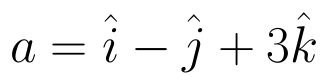and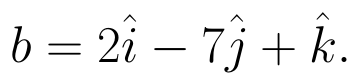(View Answer Video)

Question 2 : Find the angle between two vectors a and b with magnitudes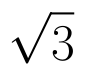and 2 respectively, having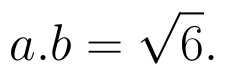(View Answer Video)

Question 3 :  If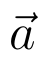and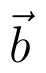denote the position vectors of points A and B respectively and C is a point on AB such that AC = 2 CB, then write the position vector of C.  (View Answer Video)

Question 4 : Find the area of the triangle with vertices A(1, 1, 2), B(2, 3, 5) and C(1, 5, 5). (View Answer Video)

Question 5 :  Find the sum of the vectors :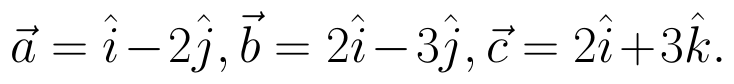(View Answer Video)

### Relations and Functions

Question 1 : Functions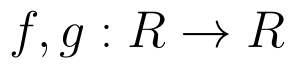are defined respectively, by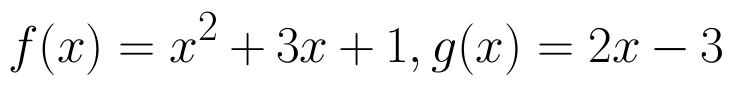, find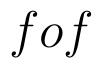(View Answer Video)

Question 2 : If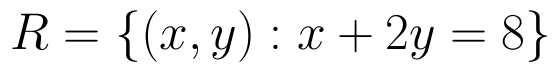is a relation on N, write the range of R. (View Answer Video)

Question 3 : If the mapping f and g are given by f = {(1, 2), (3, 5), (4, 1)} and g = {(2, 3), (5, 1), (1, 3)}, Find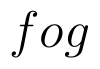. (View Answer Video)

Question 4 : Let * be the binary operation on N given by a * b = LCM of a and b. Find 5 * 7. (View Answer Video)

### Three Dimensional Geometry

Question 2 : Find the equation of the plane passing through the line of intersection of the planes x + y + z = 1 and 2x + 3y + 4z = 5 which is perpendicular to the plane x - y + z = 0. Also, find the distance of the plane obtained above from the origin. (View Answer Video)

Question 3 : A line passes through the point with position vector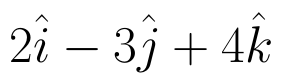and makes angles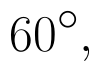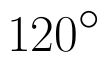and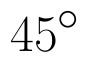with x, y and z-axis respectively. Find the equation of the line in the Cartesian form. (View Answer Video)

Question 4 : If the cartesian equations of a line are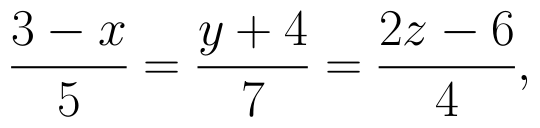write the vector equation for the line. (View Answer Video)

Question 5 : Find the vector and cartesian equations of the plane passing through the point (-1, 3, 2) and perpendicular to each of the planes x + 2y +3z = 5 and

### Inverse Trigonometric Functions

Question 1 : If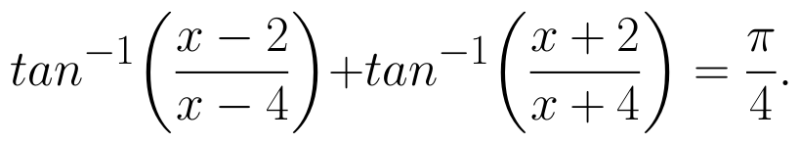Find the values of x. (View Answer Video)

Question 2 : Write the principal value of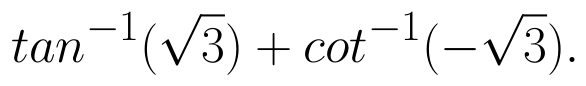(View Answer Video)

Question 3 : Evaluate :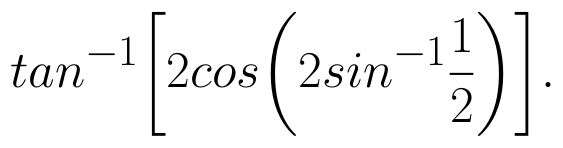(View Answer Video)

Question 4 : Write the principal value of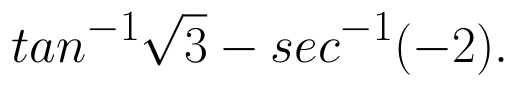(View Answer Video)

Question 5 : Solve for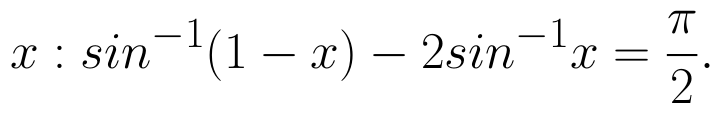(View Answer Video)